Sponsored Link •1 reply on 1 page. Most recent reply: Mar 15, 2004 8:59 PM by Coolest Head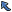Back to Topic List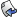Reply to this Topic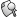Search Forum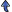Threaded View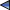Previous Topic Next Topic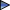Flat View: This topic has 1 reply on 1 page
 Nancy Posts: 11 Nickname: ac121107 Registered: Feb, 2004
please help get compiler error Posted: Mar 15, 2004 11:25 AMReply
 AdvertisementMy assignmsent is to write a program that roll 3 dice and repeat it 60000 times, sum the faces ,determines the frequency for each sum and the percentages. Totals the frequency and the prcentages.
Problems: Frequencies are not display in the applet. I wrote another piece of code and compiler says modifyArray(int) in Lab5 cannot be applied to modifyArray();
I was trying to modify the frequency array to obtain the percentages.
Any help is greatly appreciated
Thanks
Nancy
```// Lab5.java
// Applet that simulates rolling of 3 dice, sum the faces, display the frequency in percentages and total both the
// frequency and the percentages

import javax.swing.*;
import java.awt.*;
import java.awt.Container;
public class Lab5 extends JApplet {

JTextArea outputArea;
int face,frequency[] = new int[ 19 ];
String output= "";
double percent = 0.00;

public void init() 	{
outputArea = new JTextArea();
Container c = getContentPane();
outputArea.setFont(new Font("Courier", Font.ITALIC, 18));

showArray();
modifyArray();
}
public int rollDice()   {
int die1, die2, die3;

//roll dice 60000 times
for ( int roll= 1; roll <= 60000; roll++) {
die1 = 1 +(int) ( Math.random()  * 6 );
die2 = 1 +(int) ( Math.random()  * 6 );
die3 = 1 +(int) ( Math.random()  * 6 );

face = die1+die2+die3;
}
// use face value as subscript for frequency array
++frequency[ face ];
return face;
}

public void showArray()  {
// append frequency to String output
for (face=3; face < frequency.length; face++ ){
output +="\n" + face + "\t" + frequency[face]+ "\t\t" + percent;

outputArea.setText("Sum\tFrequency\t%"+"\n" + output );//+ "\n\n" + "Total");
}
}

public void modifyArray(int frequency)   {
percent =  frequency/600;

}

}
```

 Coolest Head Posts: 5 Nickname: stkovrflow Registered: Mar, 2004
Re: please help get compiler error Posted: Mar 15, 2004 8:59 PMReply
> My assignmsent is to write a program that roll 3 dice and
> repeat it 60000 times, sum the faces ,determines the
> frequency for each sum and the percentages. Totals the
> frequency and the prcentages.
> Problems: Frequencies are not display in the applet. I
> wrote another piece of code and compiler says
> modifyArray(int) in Lab5 cannot be applied to
> modifyArray();
> I was trying to modify the frequency array to obtain the
> percentages.
> Any help is greatly appreciated
> Thanks
> Nancy
>
```> // Lab5.java
> // Applet that simulates rolling of 3 dice, sum the faces,
> display the frequency in percentages and total both the
> // frequency and the percentages
>
> import javax.swing.*;
> import java.awt.*;
> import java.awt.Container;
> public class Lab5 extends JApplet {
>
>     JTextArea outputArea;
> 	int face,frequency[] = new int[ 19 ];
> 	String output= "";
> 	double percent = 0.00;
>
> 	public void init() 	{
> 	    outputArea = new JTextArea();
> 		Container c = getContentPane();
> outputArea.setFont(new Font("Courier", Font.ITALIC,
> C, 18));
>
> 		showArray();
>[u][b]		modifyArray(); [/b][/u]
> 	}
> 	public int rollDice()   {
> 		int die1, die2, die3;
>
> 	    //roll dice 60000 times
> 		for ( int roll= 1; roll <= 60000; roll++) {
> 	    die1 = 1 +(int) ( Math.random()  * 6 );
> 		die2 = 1 +(int) ( Math.random()  * 6 );
> 	    die3 = 1 +(int) ( Math.random()  * 6 );
>
> 	    face = die1+die2+die3;
>         }
> 	    // use face value as subscript for frequency array
> 		++frequency[ face ];
> 		return face;
> 	}
>
> 	public void showArray()  {
>     // append frequency to String output
> 	for (face=3; face < frequency.length; face++ ){
> output +="\n" + face + "\t" + frequency[face]+ "\t\t" +
> + percent;
>
> outputArea.setText("Sum\tFrequency\t%"+"\n" + output
> tput );//+ "\n\n" + "Total");
> 	}
>     }
>
>     public void modifyArray(int frequency)   {
> 	   percent =  frequency/600;
>
>
>     }
>
>
> }
>
```

Look at where you are calling modifyArray(). You are calling it without parameters and yet your function definition has a int parameter. Get the idea???

 Flat View: This topic has 1 reply on 1 pagePrevious Topic Next TopicWeb Artima.com